## Syntax

A formula in Google Sheets is used to do mathematical calculations. Formulas always start with the equal sign `=` typed in the cell, followed by your calculation.

Note: You claim the cell by selecting it and typing the equal sign (`=`)

### Creating formulas, step by step

• Select a cell
• Type the equal sign (`=`)
• Select a cell or type a value
• Enter an arithmetic operator
• Select another cell or a type value
• Press Enter

For example `=1+1` is the formula to calculate 1+1=2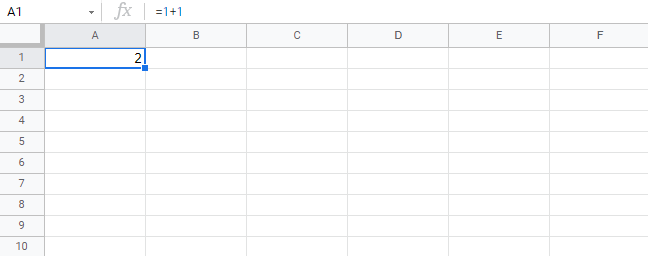Note: The value of a cell is communicated by reference(value) for example `A1(2)` means the cell `A1` has value `2`.

## Using Formulas with Cells

You can type values to cells and use them in your formulas.

Lets type some dummy values to get started. Double click the cells to type values into them. Go ahead and type:

• `A1(309)`
• `A2(320)`
• `B1(39)`
• `B2(35)`

Compare with the picture shown below: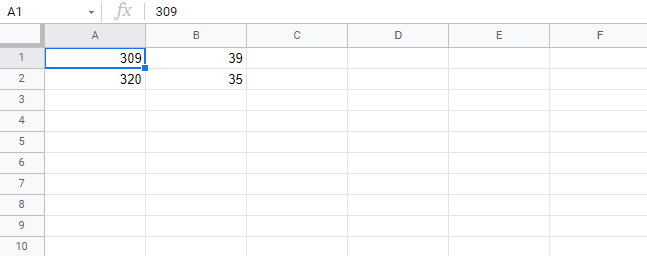Copy Values

Note: Type values by selecting a cell, claim it by entering the equal sign (`=`) and then type your value. For example `=309`.

Well done! You have successfully typed values to cells and now we can use them to create formulas.

Here is how to do it, step by step.

1. Select the cell `C1`
2. Type the equal sign (`=`)
3. Select `A1`, the cell that has the `(309)` value
4. Type the minus sign (`-`)
5. Select `B2`, the cell that has the `(35)` value
6. Hit enter

Tip: The formula can be typed directly without clicking the cells. The typed formula would be the same as the value in `C1` `(=A1-B2)`.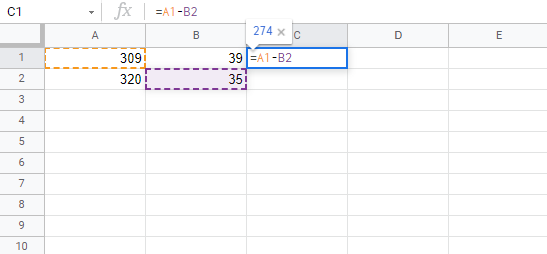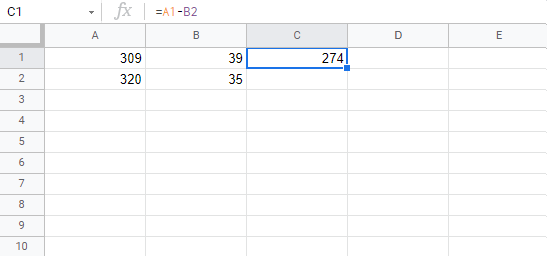The result after hitting the Enter button is `C1(274)`. Did you make it?

## Another Example

Let's try one more example, this time let's make the formula `=A2-B1`.

Here is how to do it, step by step.

1. Select the cell `C2`
2. Type the equal sign (`=`)
3. Select `A2`, the cell that has the `(320)` value
4. Type the minus sign (`-`)
5. Select `B1`, the cell that has the `(39)` value
6. Hit the Enter button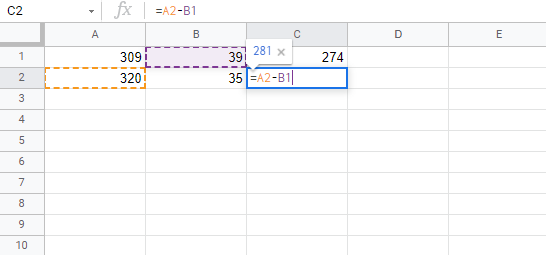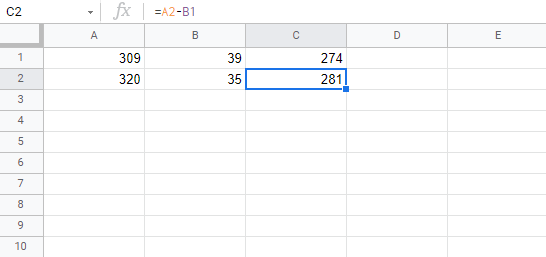You got the result `C2(281)`, right? Way to go!

Note: You can make formulas with all four arithmetic operations, such as addition (`+`), subtraction (`-`), multiplication (`*`) and division (`/`).

Here are some examples:

• `=2+4 gives you 6`
• `=4-2 gives you 2`
• `=2*4 gives you 8`
• `=2/4 gives you 0.5`

In the next chapter you will learn about Ranges and how data can be moved in the Sheet.

W3Schools is optimized for learning and training. Examples might be simplified to improve reading and learning. Tutorials, references, and examples are constantly reviewed to avoid errors, but we cannot warrant full correctness of all content. While using W3Schools, you agree to have read and accepted our terms of use, cookie and privacy policy.